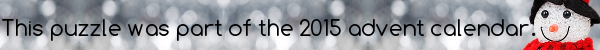mscroggs.co.ukmscroggs.co.uksubscribe

# Puzzles

## Not Roman numerals

The letters $$I$$, $$V$$ and $$X$$ each represent a different digit from 1 to 9. If
$$VI\times X=VVV,$$
what are $$I$$, $$V$$ and $$X$$?

## Backwards fours

If A, B, C, D and E are all unique digits, what values would work with the following equation?
$$ABCCDE\times 4 = EDCCBA$$

## 10 DecemberHow many zeros does 1000! (ie 1000 × 999 × 998 × ... × 1) end with?

## 17 DecemberIn March, I posted the puzzle One Hundred Factorial, which asked how many zeros 100! ends with.
What is the smallest number, n, such that n! ends with 50 zeros?

## One hundred factorial

How many zeros does $$100!$$ end with?

## Archive

Show me a random puzzle
▼ show ▼